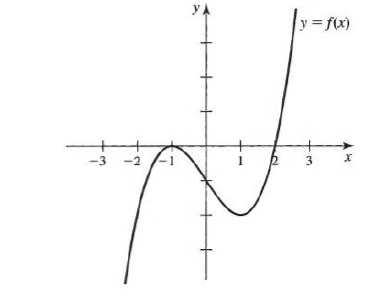×
Get Full Access to Calculus: Early Transcendentals - 1 Edition - Chapter 1.3 - Problem 11e
Get Full Access to Calculus: Early Transcendentals - 1 Edition - Chapter 1.3 - Problem 11e

×

# Solved: One-to-one functions Answer the questions in theISBN: 9780321570567 2

## Solution for problem 11E Chapter 1.3

Calculus: Early Transcendentals | 1st Edition

• Textbook Solutions
• 2901 Step-by-step solutions solved by professors and subject experts
• Get 24/7 help from StudySoup virtual teaching assistantsCalculus: Early Transcendentals | 1st Edition

4 5 1 365 Reviews
10
0
Problem 11E

One-to-one functions: Answer the questions in the following exercises using the graph of f.

Find three intervals on which f is one-to-one, making each interval as large as possible.Step-by-Step Solution:

Step 1 of 3

Given,We need to find three intervals on which f is one-to-one, making each interval as large as possible.

Step 2 of 3

Step 3 of 3

##### ISBN: 9780321570567

Unlock Textbook Solution